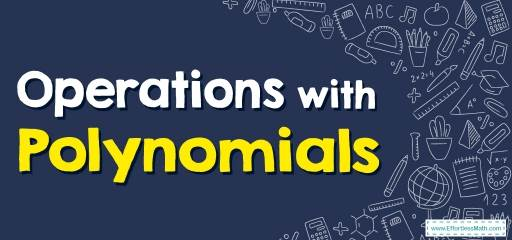# How to Do Operations with Polynomials? (+FREE Worksheet!)

Do you want to know how to solve Operations with Polynomials? you can do it in one easy steps.## Step by step guide to do Operations with Polynomials

• When multiplying a monomial by a polynomial, use the distributive property.
$$\color{blue}{a×(b+c)=a×b+a×c}$$
• For adding and subtracting polynomials remember to find and combine like terms.

The Absolute Best Book to Ace Algebra I

### Operations with Polynomials – Example 1:

Multiply. $$4(3x-5)-4x=$$

Solution:

Use the distributive property: $$\color{blue}{a×(b+c)=a×b+a×c}$$

Then: $$4(3x−5)= 12x−20$$

Now, combine like terms: $$12x-4x=8x$$

Then simplify: $$4(3x-5)-4x=12x-20-4x=8x-20$$

### Operations with Polynomials – Example 2:

Multiply. $$6x(3x+7)+12x-x^2=$$

Solution:

Use the distributive property: $$\color{blue}{a×(b+c)=a×b+a×c}$$

Then: $$6x(3x+7)=18x^2+42x$$

Now, combine like terms: $$18x^2-x^2=17x^2$$ , $$42x+12x=54x$$

Then simplify: $$6x(3x+7)+12x-x^2= 18x^2+42x+12x-x^2=17x^2+54x$$

### Operations with Polynomials – Example 3:

Multiply. $$5(2x-6)-x^2+4x=$$

Solution:

Use the distributive property: $$\color{blue}{a×(b+c)=a×b+a×c}$$

Then: $$5(2x-6)=10x-30$$

Now, combine like terms: $$10x+4x=14x$$

Then simplify: $$5(2x-6)-x^2+4x= 10x-30 -x^2+4x=-x^2+14x-30$$

The Absolute Best Book to Ace Pre-Algebra

### Operations with Polynomials – Example 4:

Multiply. $$2x(6x+2)=$$

Solution:

Use the distributive property: $$\color{blue}{a×(b+c)=a×b+a×c}$$

Then: $$2x(6x+2)=12x^2+4x$$

## Exercises for Solving Operations with Polynomials

### Find each product.

1. $$\color{blue}{3x^2 (6x – 5)+12x}$$
2. $$\color{ blue }{5x^2 (7x – 2)-x^2}$$
3. $$\color{blue}{– 3 (8x – 3)+5x}$$
4. $$\color{blue}{6x^3 (– 3x + 4)-2x^2}$$
5. $$\color{blue}{9 (6x + 2)+14x+8}$$
6. $$\color{blue}{8 (3x + 7)-x^4}$$

1. $$\color{blue}{18x^3 – 15x^2+12x}$$
2. $$\color{ blue }{35x^3 – 11x^2}$$
3. $$\color{blue}{–19x + 9}$$
4. $$\color{blue}{–18x^4 + 24x^3-2x^2}$$
5. $$\color{blue}{68x + 26}$$
6. $$\color{blue}{-x^4+24x + 56}$$

The Best Books to Ace Algebra

### What people say about "How to Do Operations with Polynomials? (+FREE Worksheet!) - Effortless Math: We Help Students Learn to LOVE Mathematics"?

No one replied yet.

X
45% OFF

Limited time only!

Save Over 45%

SAVE $40 It was$89.99 now it is \$49.99